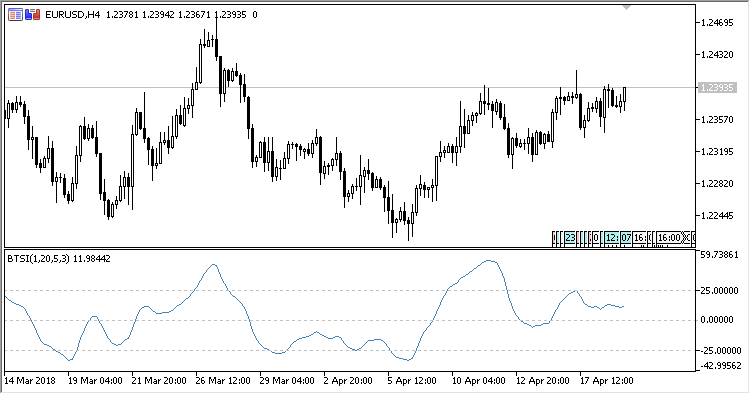# Blau_TSI – indicator for MetaTrader 5

• A+
category：MT5 INDICATORS

The William Blau True Strength Index indicator shows the William Blau true strength index.

It has seven input parameters:

• Period - calculation period;
• Applied price - the calculation price;
• First smoothing period - the initial smoothin period;
• Second smoothing period - the secondary smoothing period;
• Third smoothing period - the resulting smoothing period;
• Upper level - the overbought level;
• Lower level - the oversold level.

Based on the True Strength Index calculation formula described in the MQL5: Create your own indicator article and on William Blau's postulate of three-period smoothing, the indicator calculation formula is as follows:

`TSI(Price,Period,fs,ss,ts) = 100 * EMA(EMA(EMA( mtm(Price,Period) ,fs),ss),ts) / EMA(EMA(EMA( |mtm(Price,Period)| ,fs),ss),ts)`

where:

```mtm(Price,Period) = Price[i] - Price[i-Period+1],
fs - First smoothing period,
ss - Second smoothing period,
ts - Third smoothing period
```